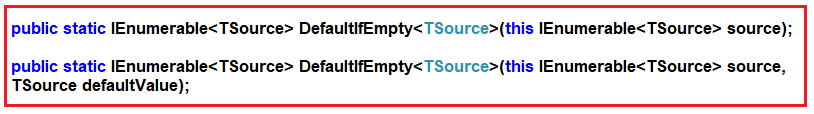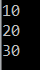# DefaultIfEmpty Method in Linq

## DefaultIfEmpty Method in Linq

In this article, I am going to discuss the DefaultIfEmpty Method in Linq with some examples. Please read our previous article before proceeding to this article, where we discussed the Single and SingleOrDefault method with some examples.

#### Linq DefaultIfEmpty Method:

If the sequence or data source on which the DefaultIfEmpty method is called is not empty, then the values of the original sequence or data source are going to be returned. On the other hand, if the sequence or data source is empty, then it returns a sequence with the default values based on the data type.

There are two overloaded versions available for this DefaultIfEmpty method in Linq which are shown in the below image.The first overloaded version does not take any parameter and in this case, if the sequence is empty then it will return the default values based on the data type.

In the second overloaded version of the DefaultIfEmpty method, you can pass the default value and if the sequence is empty then this default value (what you pass to the method) is going to be returned by the method.

##### Example1:

In the following example, the sequence is not empty. So, it is going to return a copy of the original values.

```using System.Linq;
using System;
using System.Collections.Generic;
namespace LINQDemo
{
class Program
{
static void Main(string[] args)
{
List<int> numbers = new List<int>() {10, 20, 30};
IEnumerable<int> result = numbers.DefaultIfEmpty();
foreach (int num in result)
{
Console.WriteLine(num);
}
}
}
}
```

Output:##### Example2:

In the below example, the sequence is empty. So, in this case, it is going to return “0” as the default value. This is because 0 is the default value for the integer data type.

```using System.Linq;
using System;
using System.Collections.Generic;
namespace LINQDemo
{
class Program
{
static void Main(string[] args)
{
List<int> numbers = new List<int>() {};
IEnumerable<int> result = numbers.DefaultIfEmpty();
foreach (int num in result)
{
Console.WriteLine(num);
}
}
}
}
```

Output: 0

##### Example3:

In the following example, the sequence is empty but here we have supplied a default value (i.e. 5) to the DefaultIfEmpty method. So, in this case, the default value that we supplied (5) is going to be returned.

```using System.Linq;
using System;
using System.Collections.Generic;
namespace LINQDemo
{
class Program
{
static void Main(string[] args)
{
List<int> numbers = new List<int>() {};
IEnumerable<int> result = numbers.DefaultIfEmpty(5);
foreach (int num in result)
{
Console.WriteLine(num);
}
}
}
}
```

Output: 5

Note: If we supplied a default value but the sequence is not empty then, in that case, the original values that are present in the sequence is going to be returned.

##### Example4:

In the below example, we have supplied a default value i.e. 5 to the DefaultIfEmpty method but the sequence is not empty. So, in this case, the elements which are present in the sequence are going to be returned.

```using System.Linq;
using System;
using System.Collections.Generic;
namespace LINQDemo
{
class Program
{
static void Main(string[] args)
{
List<int> numbers = new List<int>() {10, 20, 30};
IEnumerable<int> result = numbers.DefaultIfEmpty(5);
foreach (int num in result)
{
Console.WriteLine(num);
}